Next: Persistent Behaviors Up: PARTIAL FORMALIZATIONS AND THE Previous: Geography of Game7

# Predicting the Future

Prediction is difficult--especially of the future.

We use situation calculus.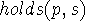means that the proposition (i.e. propositional fluent)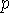holds in situation.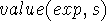is the value of the expression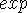in situation.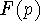is the propositional fluent that propositionwill hold in the future.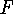is a reified temporal logic operator. We usually omit parentheses for functions of one argument so that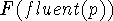would be written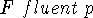.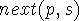is the next situation afterin whichholds. It is related toby the axiom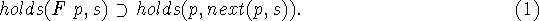The function F plays the role of a temporal logic future operator. next(p,s) denotes the next situation after s in which p holds. We intend to say nothing about the value of next(p,s) when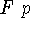doesn't hold.

[An alternative is to regard next(p,s) as an individual concept as discussed in [McCarthy, 1979b]. We would then write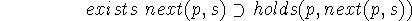as the axiom.]

We also have a conditional future function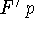. The intent is that it predicts that p will hold if nothing to prevent it happens. There will be an axiom of the form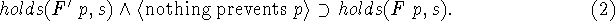It isn't yet clear how to write the condition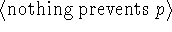.

Here is one way.

What will surely occur is any p such that F'(p,s) and such that there is no p' occurring earlier and preventing p. Therefore, we have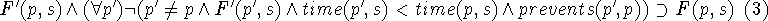We can put this in a form that is less explicit about time, namely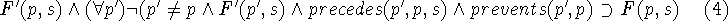Another possibility is to consider the fluents p as having associated times or at least a temporal order. This makes them richer objects than we have previously taken them to be. The equations then become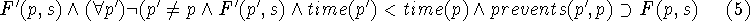and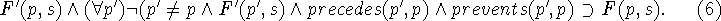Probably the basic inference task is predicting what happens in a situation when there is no intervention. This is just predicting what would be observed by running the program, but predictions used for planning need to be done in an epistemologically adequate way, i.e. using only the information that would be available to a human player. The result will be qualitative, sometimes uncertain and sometimes incorrect.

The basic fact about lemmings walking can be formulated in first order logic as follows.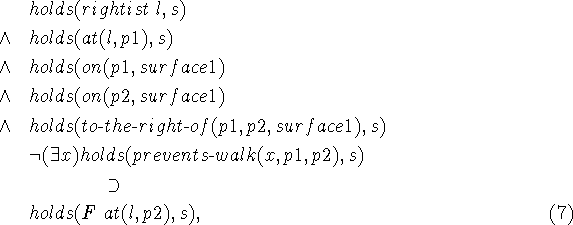where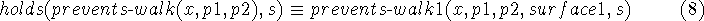and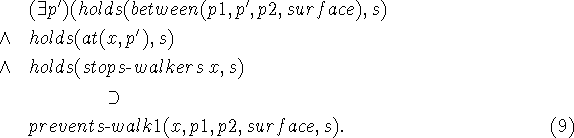We have made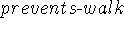a term so it can be an argument of holds, whereas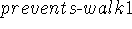is a predicate so it can be circumscribed. Maybe there will be some way to get rid of this bureaucratic complication.

We would circumscribe the predicate, and if there were no obstacle, we would be able to infer that lemming l would indeed reach point p2.

Additional phenomena that might prevent walking to a destination are to be treated by adding sentences whose conclusion is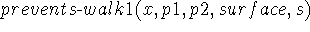, i.e. the conclusion of (9).

The above equations are unpleasantly long, and in more complicated cases might get quite a bit longer. In dealing with situations that actually arise in playing a game, these equations would be paralleled by a program that would establish that lemming l would reach p2 in the concrete situation. In subsection 8.2 we discuss how a program can be connected to a predicate or function in such a way that when it is necessary to reason logically about the predicate applied to constant arguments the program can be used to get the value. We call this attachment of the predicate to the program. It is a special case of partial evaluation.

We might hope to use program in more abstract cases, e.g. thinking about hypothetical situations, like ``if I had a blocker there''. Constant symbols and construction functions would be needed to construct the a representation of the hypthetical situation itself and the other constants on which the program would operate. Presumably the hypthetical situations would be objects in the sense of object-oriented languages, e.g. CLOS.

However, only equations retain elaboration tolerance, e.g. permit the introduction of new lemming properties by making an assertion.Next: Persistent Behaviors Up: PARTIAL FORMALIZATIONS AND THE Previous: Geography of Game7

John McCarthy
Mon Mar 2 16:21:50 PDT 1998